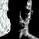# UCS_S_Steve Primo - Strategy 4 - Version 2

Dilihat 10493 kali
For those who are interested with this strategy, Here is a better version.
```// Created by UCSgears
// Includes options to confirm with Pet-d

study(shorttitle="SP-S#4", title = "Steve Primo - Strategy #4", overlay = true, precision = 2)

basisma = sma(close,50)
petd = ema(close,15)

usepetd = input(true, title = "PET-D Confirmation Required")

trendup = usepetd == 1 ? close > basisma and close > petd : close > basisma
trenddn = usepetd == 1 ? close < basisma and close < petd : close < basisma

// PET-D

petdcolor = close > petd ? green : red
barcolor (petdcolor)

// Pullback & Bounce Criteria
lowest = lowest(low,(5))
highest = highest(high,(5))
pullback = (low == lowest) or (low == lowest) ? 1 : 0
bounce = (high == highest) or (high == highest) ? 1 : 0

// ALL PLOT
plot (lowest, color = green, linewidth = 1, title = "Lower Band")
plot (highest, color = red, linewidth = 1, title = "Upper Band")
plot (basisma, color = black, linewidth = 3, title = "Trend - Long Term")
plot (petd, color = blue, linewidth = 1, title = "Trend - Short Term")

// 25% Close
range = high - low
rbrng = abs(open-close)
// Long Setup
longcandle = close > low+range*.75 ? 1:0
// Short Setup
shortcandle = close < low+range*.25 ? 1:0

//Setups
setuplong = trendup == 1 and pullback == 1 and longcandle == 1 ? 1:0
setupshort = trenddn == 1 and bounce == 1 and shortcandle == 1 ? 1:0

//setuplong = setuplonggeneral == 1 and setuplonggeneral == 0 ? 1:0
//setupshort = setupshortgeneral == 1 and setupshortgeneral == 0 ? 1:0

bcl = rbrng > (rma(tr,5)*1.1) ? blue : green
bcs = rbrng > (rma(tr,5)*1.1) ? blue : red

plotchar(setuplong, title="Long Setup Bar", char='⇑', location=location.belowbar, color=bcl, transp=0, text="Strategy #4 Long")
plotchar(setupshort, title="Short Setup Bar", char='⇓', location=location.abovebar, color=bcs, transp=0, text="Strategy #4 Short")

tiggerlongcandle = (setuplong == 1) and (high > high) ? 1 : 0
tiggershortcandle = (setupshort == 1) and (low < low) ? 1 : 0
plotshape(tiggerlongcandle ? tiggerlongcandle : na, title="Triggered Long",style=shape.triangleup, location=location.belowbar, color=green, transp=0, offset=0)
plotshape(tiggershortcandle ? tiggershortcandle : na, title="Triggered Short",style=shape.triangledown, location=location.abovebar, color=red, transp=0, offset=0)

tarup = (tiggerlongcandle==1 and setuplong == 1) ? high+range : na
plotshape(tarup, style = shape.circle, location = location.absolute, title = "Target Long", color = white)

tardn = (tiggershortcandle==1 and setupshort == 1) ? low-range : na
plotshape(tardn, style = shape.circle, location = location.absolute, title = "Target Short", color = white)
```

## KomenWow. You are really prolific.

I am not a programmer, so I got one question for you.

Format-Style:
Long setup bar
"Color 0" means dont enter the trade
and
"Color 1" means enter the trade
?
BalasCryptoFxWorks
I cannot give the MT4 code,Steve Primo is directly selling, Just to avoid any legal litigation, I would avoid that scenario...... Any the concept is here, feel free to write the code.... Sorry.
Balasmeleysr.1
replace the // with #.

The code needs to be modified a bit before it can be used at tos
Balas